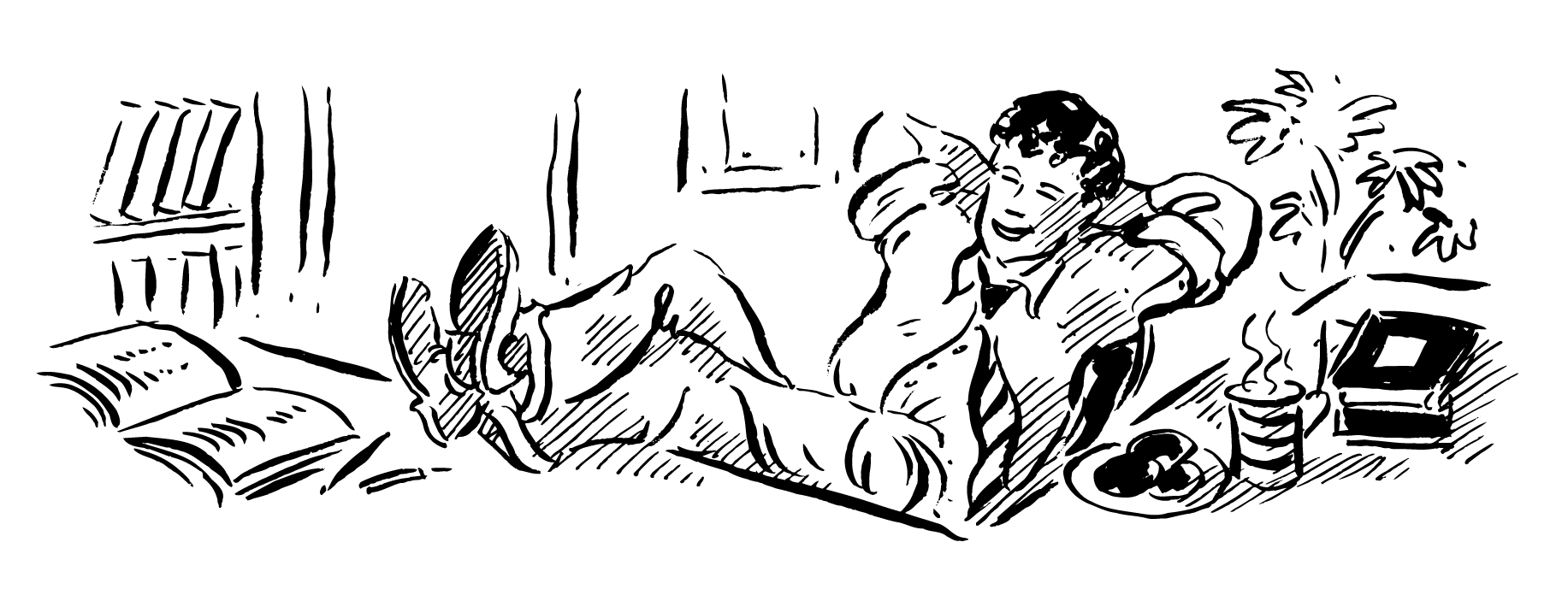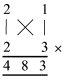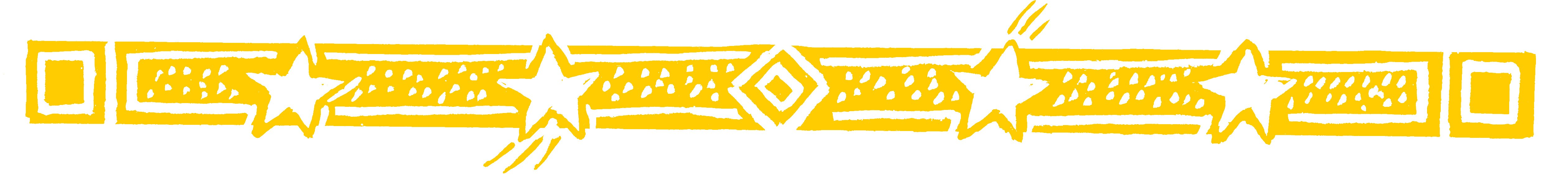HAVE A BREAKAt break time at school or in the office try out this elegant way of multiplying numbers using a simple pattern.

• 21 x 23 = 483

This is normally called long multiplication but
actually the answer can be written straight down
using the VERTICALLY AND CROSSWISE
formula.

We first put, or imagine, 23 below 21There are 3 steps:

a) Multiply vertically on the left: 2 x 2 = 4
This gives the first figure of the answer.

b) Multiply crosswise and add: 2 x 3 + 1 x 2 = 8
This gives the middle figure.

c) Multiply vertically on the right: 1 x 3 = 3
This gives the last figure of the answer.

And thats all there is to it.

•  Similarly 61 x 31 = 1891• 6 x 3 = 18; 6 x 1 + 1 x 3 = 9; 1 x 1 = 1

Question Comment !
 Tutorial Title Question Title No Questions 0 Questions Asked 0 Skipped Questions 0 Correctly Answered 0 Wrongly Answered 0 Total Question Attempts 0 Current Question Attempts 0

Skip Question

Reset Test

Halt Test

 No Questions 0 Questions Asked 0 Skipped Questions 0 Correctly Answered 0 Wrongly Answered 0 Total Question Attempts 0

Time Taken

CloseHave A BreakThe creative principle
resides in mathematics
EINSTEIN